# Help inspire your kindergartner to become an outside-the-box thinker.

Our Kindergarten brain games align with the Common Core State Standards. Kids practice both reading and writing skills and meet many Kindergarten Curriculum standards. CLICK HERE if you are looking for PRE-K Curriculum Standards.

While playing the games, kids learn kindergarten (and some first grade) math and reading skills. Use the games to review kindergarten curriculum skills with your child or as a way to introduce new ones.

Your kids will love learning with Blimey Box Brain Games.

# Kindergarten Game Pack #1

• Kindergarten Dolch sight words
• Phonics: Beginning letter sounds
• Phonics: Ending letter sounds
• Phonics: middle letter sounds
• Alphabetical order
• Matching lowercase to uppercase letters
• Decoding a secret message

## Math skills:

• Counting by 2's
• Counting objects in random order
• Sequencing numbers to 100
• Tally marks
• Subtraction with pictures
• Find the number that makes ten
• Find the missing number
• Counting by fives
• Measuring with paperclips

## Kindergarten Prep Game Pack #1 Sample Skill Tasks: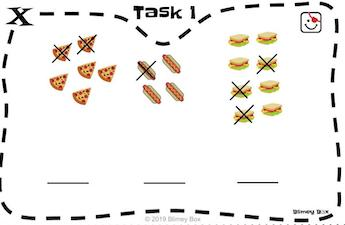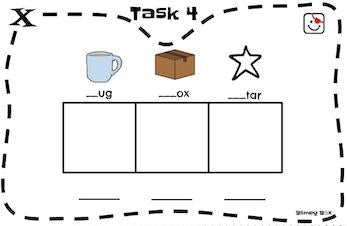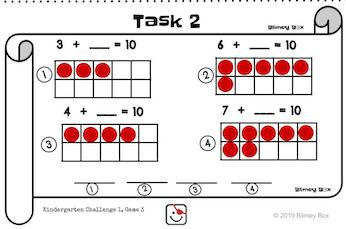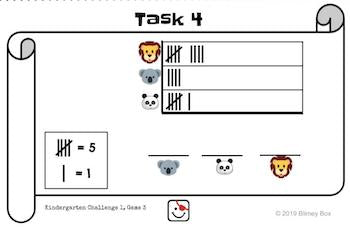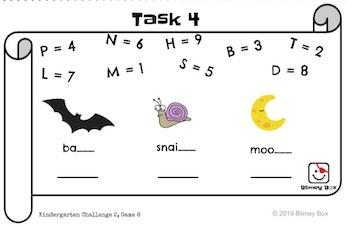# Kindergarten Game Pack #2

###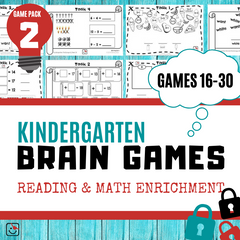• Kindergarten Dolch sight words
• Decoding a secret message
• Phonics: beginning word blends
• Sorting vowels and consonants
• Building simple sentences
• Spelling CVC words

## Math Skills:

• One-to-one number correspondence
• 2D and 3D Shape recognition
• Sorting 3D Shapes
• Counting by tens
• Combine different shapes to create a picture
• Patterning
• Building numbers to 20
• Greater than and Less than
• Addition and subtraction to 10

## Kindergarten Prep Game Pack #2 Sample Skill Tasks: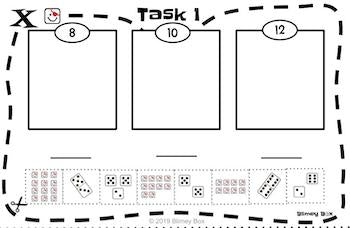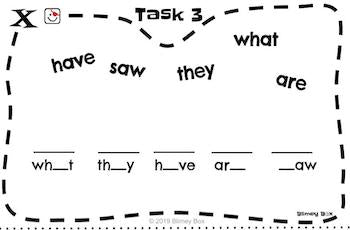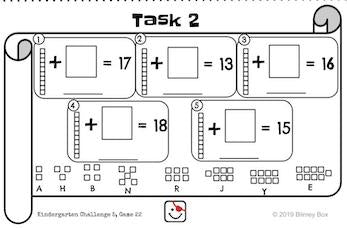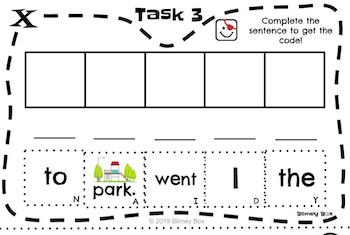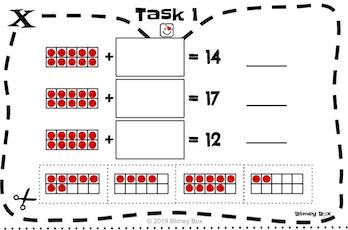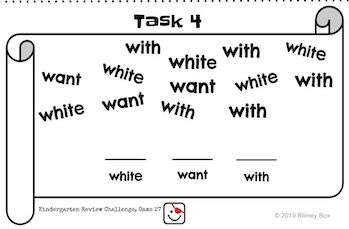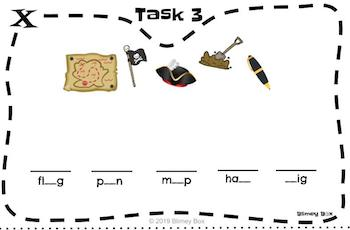Math and reading concepts spiral throughout for continued practice. And kids must apply prior knowledge to new situations to solve new tasks.

## KINDERGARTEN COMMON CORE STATE STANDARDS COVERED BY BLIMEY BOX

The Kindergarten Brain Games cover many Kindergarten curriculum standards found in the Common Core State Standards. We designed the games to help kids practice and master new skills.

## English Language Arts

Print Concepts:

CCSS.ELA-LITERACY.RF.K.1
Demonstrate understanding of the organization and basic features of print.

Phonological Awareness:

CCSS.ELA-LITERACY.RF.K.2
Demonstrate understanding of spoken words, syllables, and sounds (phonemes).

Phonics and Word Recognition:

CCSS.ELA-LITERACY.RF.K.3
Know and apply grade-level phonics and word analysis skills in decoding words.

• CCSS.ELA-LITERACY.RF.K.3.A
Demonstrate basic knowledge of one-to-one letter-sound correspondences by producing the primary sound or many of the most frequent sounds for each consonant.
• CCSS.ELA-LITERACY.RF.K.3.B
Associate the long and short sounds with the common spellings (graphemes) for the five major vowels.
• CCSS.ELA-LITERACY.RF.K.3.C
Read common high-frequency words by sight (e.g., theoftoyoushemyisaredodoes).
• CCSS.ELA-LITERACY.RF.K.3.D
Distinguish between similarly spelled words by identifying the sounds of the letters that differ.

Fluency:

CCSS.ELA-LITERACY.RF.K.4

Conventions of Standard English:

CCSS.ELA-LITERACY.L.K.1
Demonstrate command of the conventions of standard English grammar and usage when writing or speaking.

CCSS.ELA-LITERACY.L.K.2
Demonstrate command of the conventions of standard English capitalization, punctuation, and spelling when writing.

## Math standards covered in Blimey Box Brain Games:

Counting and Cardinality

#### Know number names and the count sequence.

CCSS.MATH.CONTENT.K.CC.A.1
Count to 100 by ones and by tens.

CCSS.MATH.CONTENT.K.CC.A.2
Count forward beginning from a given number within the known sequence (instead of having to begin at 1).

CCSS.MATH.CONTENT.K.CC.A.3
Write numbers from 0 to 20. Represent a number of objects with a written numeral 0-20 (with 0 representing a count of no objects).

#### Count to tell the number of objects.

CCSS.MATH.CONTENT.K.CC.B.4
Understand the relationship between numbers and quantities; connect counting to cardinality.

• CCSS.MATH.CONTENT.K.CC.B.4.A
When counting objects, say the number names in the standard order, pairing each object with one and only one number name and each number name with one and only one object.
• CCSS.MATH.CONTENT.K.CC.B.4.B
Understand that the last number name said tells the number of objects counted. The number of objects is the same regardless of their arrangement or the order in which they were counted.
• CCSS.MATH.CONTENT.K.CC.B.4.C
Understand that each successive number name refers to a quantity that is one larger.

CCSS.MATH.CONTENT.K.CC.B.5
Count to answer "how many?" questions about as many as 20 things arranged in a line, a rectangular array, or a circle, or as many as 10 things in a scattered configuration; given a number from 1-20, count out that many objects.

#### Compare numbers.

CCSS.MATH.CONTENT.K.CC.C.6
Identify whether the number of objects in one group is greater than, less than, or equal to the number of objects in another group, e.g., by using matching and counting strategies.1

CCSS.MATH.CONTENT.K.CC.C.7
Compare two numbers between 1 and 10 presented as written numerals.

Operations and Algebraic Thinking

#### Understand addition as putting together and adding to, and understand subtraction as taking apart and taking from.

CCSS.MATH.CONTENT.K.OA.A.1
Represent addition and subtraction with objects, fingers, mental images, drawings1, sounds (e.g., claps), acting out situations, verbal explanations, expressions, or equations.

CCSS.MATH.CONTENT.K.OA.A.2
Solve addition and subtraction word problems, and add and subtract within 10, e.g., by using objects or drawings to represent the problem.

CCSS.MATH.CONTENT.K.OA.A.4
For any number from 1 to 9, find the number that makes 10 when added to the given number, e.g., by using objects or drawings, and record the answer with a drawing or equation.

CCSS.MATH.CONTENT.K.OA.A.5
Fluently add and subtract within 5.

Number and Operations in Base Ten

#### Work with numbers 11-19 to gain foundations for place value.

CCSS.MATH.CONTENT.K.NBT.A.1
Compose and decompose numbers from 11 to 19 into ten ones and some further ones, e.g., by using objects or drawings, and record each composition or decomposition by a drawing or equation (such as 18 = 10 + 8); understand that these numbers are composed of ten ones and one, two, three, four, five, six, seven, eight, or nine ones.

Measurement and Data

#### Describe and compare measurable attributes.

CCSS.MATH.CONTENT.K.MD.A.1
Describe measurable attributes of objects, such as length or weight. Describe several measurable attributes of a single object.

CCSS.MATH.CONTENT.K.MD.A.2
Directly compare two objects with a measurable attribute in common, to see which object has "more of"/"less of" the attribute, and describe the difference. For example, directly compare the heights of two children and describe one child as taller/shorter.

#### Classify objects and count the number of objects in each category.

CCSS.MATH.CONTENT.K.MD.B.3
Classify objects into given categories; count the numbers of objects in each category and sort the categories by count.1

Geometry

#### Identify and describe shapes.

CCSS.MATH.CONTENT.K.G.A.3
Identify shapes as two-dimensional (lying in a plane, "flat") or three-dimensional ("solid").

#### Analyze, compare, create, and compose shapes.

CCSS.MATH.CONTENT.K.G.B.4
Analyze and compare two- and three-dimensional shapes, in different sizes and orientations, using informal language to describe their similarities, differences, parts (e.g., number of sides and vertices/"corners") and other attributes (e.g., having sides of equal length).

CCSS.MATH.CONTENT.K.G.B.5
Model shapes in the world by building shapes from components (e.g., sticks and clay balls) and drawing shapes.

CCSS.MATH.CONTENT.K.G.B.6
Compose simple shapes to form larger shapes. For example, "Can you join these two triangles with full sides touching to make a rectangle?"